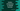# Python anonymous or lambda function : Python Tutorial 17## Python anonymous or lambda function :

Anonymous or lambda functions are functions without a name. In python, we can create an anonymous function using a construct called “lambda” unlike “def” keyword we use to create other functions.

## Difference between normal function and lambda function :

``````def function1(x) : return x ** x

function2 = lambda x : x ** x

print function1(2)
print function2(2)``````

In the above example, both print statement will give the same result “4” . The difference between both is that the lambda function does not have any return statement. In this example , we are using only one argument but lambda function can have multiple arguments.

In the above example function2 is a lambda function, “x” is its argument and “x ** x” is the return statement.

## Lambda function as a return statement :

We can also create a lambda function as return statement of other functions like :

``````def function1(x):
return lambda y : x * y

print function1(2)(3)``````

The above example will print 6.

## Lambda function with filter() :

filter() takes one list and a function as argument . Using the function, it filters out the elements from the list and returns a new list.

``````mylist = [ 1, 2, 3, 4, 5, 6, 7, 8, 9]

print filter(lambda x : x % 2 == 0 , mylist)``````

In this example, filter will pick elements from list “mylist” one by one and it will check if it is divisible by 2 or not. If divisible , it will add it to an another list. This list will be returned at last. So, the output will be : [2, 4, 6, 8]

## lambda function with map() :

map() function also takes one function and one list as argument. Similar to filter, it will return one new list. The elements of the list will be the return value for each item of the function.

``````mylist = [ 1, 2, 3, 4, 5, 6, 7, 8, 9]

print map(lambda x : x % 2 == 0 , mylist)``````

The output will be :`[False, True, False, True, False, True, False, True, False]`

## lambda function with reduce() :

reduce() takes two arguments as like the above two . But the function passes as argument should also have two arguments not one. It will calculate the result for the first two , then again it will calculate result and the third value and so on. Let’s take a look :

``````mylist = [ 1, 2, 3, 4, 5, 6, 7, 8, 9]

print reduce(lambda x,y : x + y , mylist)``````

This example will print the sum of all the elements of the list “mylist” i.e. 45.